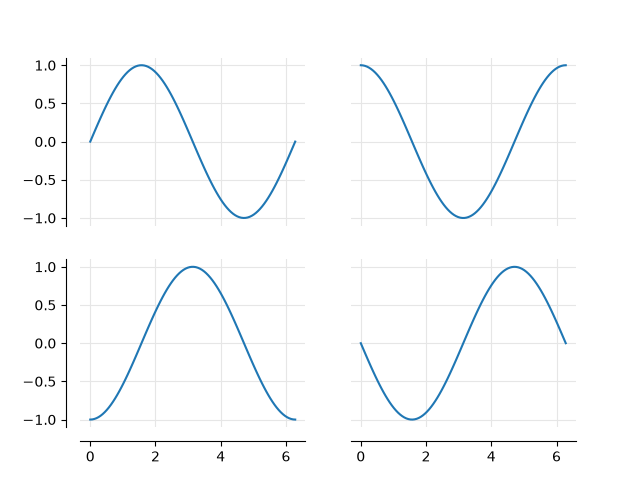# Dropped spines#

Demo of spines offset from the axes (a.k.a. "dropped spines").import matplotlib.pyplot as plt
import numpy as np

ax.label_outer(remove_inner_ticks=True)
ax.grid(color='0.9')

for loc, spine in ax.spines.items():
if loc in visible_spines:
spine.set_position(('outward', 10))  # outward by 10 points
else:
spine.set_visible(False)

x = np.linspace(0, 2 * np.pi, 100)

fig, axs = plt.subplots(2, 2)

axs[0, 0].plot(x, np.sin(x))
axs[0, 1].plot(x, np.cos(x))
axs[1, 0].plot(x, -np.cos(x))
axs[1, 1].plot(x, -np.sin(x))